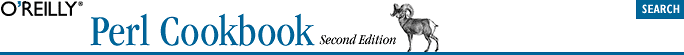home | O'Reilly's CD bookshelfs | FreeBSD | Linux | Cisco | Cisco Exam## 11.3. Taking References to Hashes

### 11.3.2. Solution

To get a hash reference:

```\$href = \%hash;
\$anon_hash = { "key1" => "value1", "key2" => "value2", ... };
\$anon_hash_copy = { %hash };```

To dereference a hash reference:

```%hash  = %\$href;
\$value = \$href->{\$key};
@slice = @\$href{\$key1, \$key2, \$key3};  # note: no arrow!
@keys  = keys %\$href;```

To check whether something is a hash reference:

```if (ref(\$someref) ne "HASH") {
die "Expected a hash reference, not \$someref\n";
}```

### 11.3.3. Discussion

This example prints out all keys and values from two predefined hashes:

```foreach \$href ( \%ENV, \%INC ) {       # OR: for \$href ( \(%ENV,%INC) ) {
foreach \$key ( keys %\$href ) {
print "\$key => \$href->{\$key}\n";
}
}```

Access slices of hashes by reference as you'd access slices of arrays by reference. For example:

```@values = @\$hash_ref{"key1", "key2", "key3"};

for \$val (@\$hash_ref{"key1", "key2", "key3"}) {
\$val += 7;   # add 7 to each value in hash slice
}```